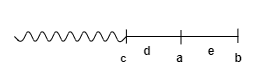# Problem: An object oscillates back and forth on the end of a spring. Which of the following statements are true at some time during the course of the motion?a. The object can have zero acceleration and, simultaneously, nonzero velocity.b. The object can have zero velocity and, simultaneously, zero acceleration.c. The object can have nonzero velocity and nonzero acceleration simultaneously.d. The object can have zero velocity and, simultaneously, nonzero acceleration.

###### FREE Expert Solution

In this problem, we are required to identify the characteristics of turning points, the equilibrium, and other intermediate positions.

Let's represented this situation using a horizontal spring, and identify the properties of the characteristic points.a is the equilibrium point, b and c are the turning points, d and e are any intermediate points.

87% (6 ratings)###### Problem Details

An object oscillates back and forth on the end of a spring. Which of the following statements are true at some time during the course of the motion?

a. The object can have zero acceleration and, simultaneously, nonzero velocity.
b. The object can have zero velocity and, simultaneously, zero acceleration.
c. The object can have nonzero velocity and nonzero acceleration simultaneously.
d. The object can have zero velocity and, simultaneously, nonzero acceleration.